# Gravitational Force Interaction in MATLAB

August 27, 2021

By  Soumya Shaw

lang: en_US

## Introduction

The Gravitational Force is one of the oldest forces that has been discovered by Physicists and is still baffling on some levels. Sir Isaac Newton was the first to define the force mathematically. He presented an analytical relation between the magnitude of the force and the masses interacting and the distance of separation between them. In his first law of motion, Newton said that force is the amount of change in momentum producing it. (i.e., a force applied to an object has an equal and opposite reaction) Hence, Gravitational force results from mass and momentum interaction according to gravitation equations as stated below.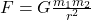Originally, gravitational force was defined by Newton as a result of two masses. It was discovered that such a situation typically did not occur in nature. Still, the realization didn't stop scientists from exploring further into this force which would eventually become known as gravitational interaction. The main problem with this theory is its inability to predict experimental results accurately when another body was brought into play. Eventually, it was discovered that an increase in the number of objects (m1, m2) involved in the equation would prove to be more accurate.

So far, there hasn't been any experimentation conducted to test the validity of this theory or any conservation of energy equations in general. How can one be able to confirm a conservation equation if they haven't conducted any experiments? The closest thing to an experiment would be observations. We can confirm that gravitational interaction exists between two bodies, which means we have confirmation about the conservation of energy for sure. However, keeping these problems for the core Physicists, we will just look upon the basic form of the equation and use it to describe motions using MATLAB.

## Simulating Gravitational Force in free space

The Gravitation Equation is an approximation that has been derived based on Newton's Law of Universal Gravitation. In Physics, Newton's Law states that each point mass attracts every other point mass by a directly proportional force to the product of their masses and inversely proportional to the square of the distance between them. The proportionality constant, the gravitational constant, in this case, has a very precise value of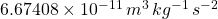devised by very sophisticated experimentations.

While two bodies in free space is a very trivial and straightforward model of Gravitational force interaction, it is fundamental in describing every other complex motion. So, we will begin our simulation journey by having a mass of 10kg fixed in spatial dimensions at a point and a free body interacting in its Gravitational field. A free-body model is one where no external forces act on it andare simply subject to its mass and gravity.

We have our object of mass 10kg and an empty space where another object of unspecified mass will be our free body. Since this is a Newtonian simulation, with every tick of time, the force applied to the mass will be equal to the Gravitational constant * mass of Earth * distance between them. Since we are not computing acceleration but using the time taken to reach an equilibrium state, we will need a function that calculates how long it takes a given mass at rest to come to rest (i.e., distance reduction).

While the formula is descriptive enough for calculations, it cannot be used directly for simulating the scenario. The formula must be converted into an ordinary differential equation (ODE) format describing the conditions or forces it depends on. So, the ODE analog of the equation is as shown below.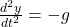where g also depends upon the masses concerned and the distance between the objects, as already mentioned before. Here is the respective MATLAB code for using the ODE for simulating the scenario described above.

clc
clear all
close all
%% Solving the Ordinary Differential Equation
G = 6.67408e-11; %Gravitational constant
M = 10; %Mass of the fixed object
r = 1; %Distance between the objects
tspan = [0 100000]; %Time Progression from 0 to 100000s
conditions = [1;0]; %y0= 1m apart, v0=0 m/s
[t,y]=ode45(@(t,y)var_r(t,y,G,M,r),tspan,conditions); %ODE solver algorithm
plot(t,y(:,1)); %Plotting the Graph
xlabel('time (s)')
ylabel('distance (m)')
%% Animation of Results
plot(0,0,'b.','MarkerSize', 20);
hold on
fori=1:length(t)
k = plot(y(i,1),0,'r.','MarkerSize', 12);
hold on
pause(0.05);
axis([-1 2 -2 2]) %Defining the Axis
xlabel('X-axis') %X-Axis Label
ylabel('Y-axis') %Y-Axis Label
delete(k)
end
function yd=var_r(t,y,G,M,r) %function of variable r
g = (G*M)/(r + y(1))^2;
yd = [y(2); -g];
end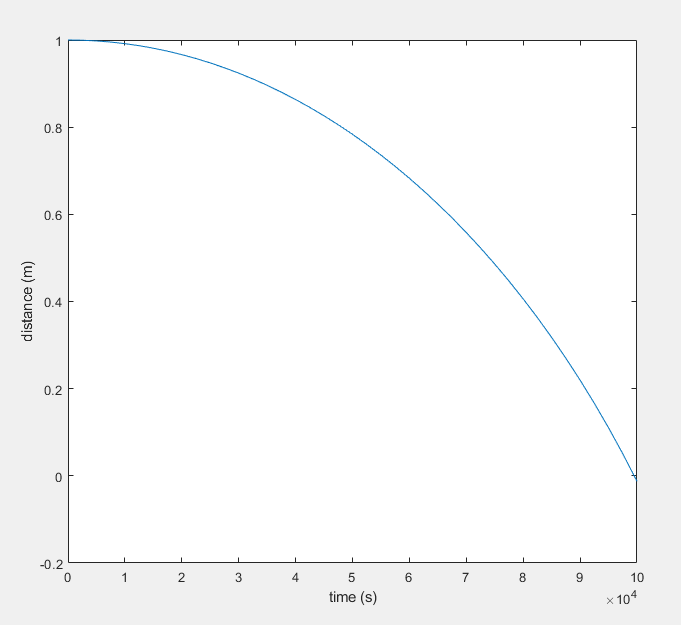Distance Vs Time graph for the Gravitational Interaction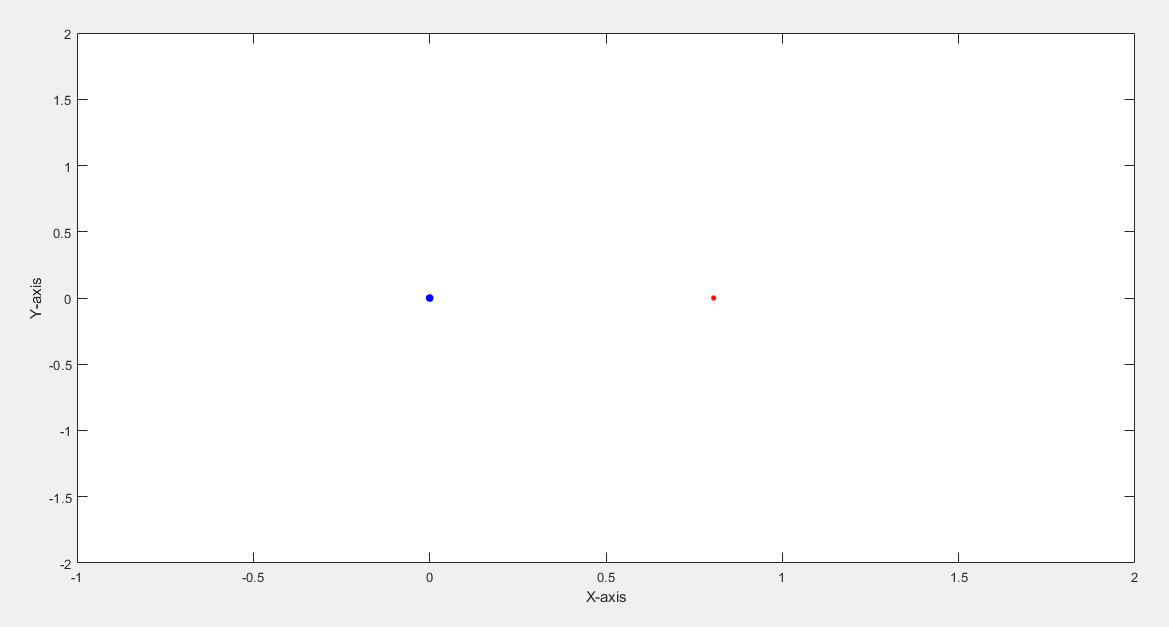A still image of the animation showing the Interaction

## Runge-Kutta Method

The solver technique you just saw in the MATLAB code must have raised many questions in your mind, like what exactly is the ode45 function, which was able to solve the equation. But for that, we need to go to the basics for a while.

Questions concerning ordinary differential equations (ODEs) may always be simplified to the study of differential equations of first order. For example, the following second-order equation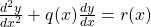can be rewritten as two first-order differential equations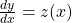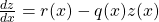where z is a new variable. Following the same trend, we can reformulate the former differential equation concerning the gravitational force into two subsequent ODEs.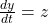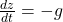The ode45 function calculates the next point in the resultant sequence with the var_r function linked to it, presenting the two ODEs after calculating the variable distance from the fixed point. Let us see how the function operates internally.

Runge-Kutta methods propagate a solution over an interval by combining the information from several Euler-style steps (each involving one evaluation of the right-hand function) and then using the information obtained to match a Taylor series expansion up to some higher order. The formula for the Euler method is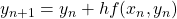which advances a solution from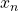to.

The formula is unsymmetrical: It advances through an interval h but uses derivative information only at the beginning of that interval.

There are many versions of the Runge Kutta method. Still, the most used version is the classical fourth-order Runge-Kutta formula, as shown below: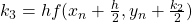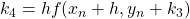The fourth-order Runge-Kutta method requires four evaluations of the right-hand side per step as shown in the following figure.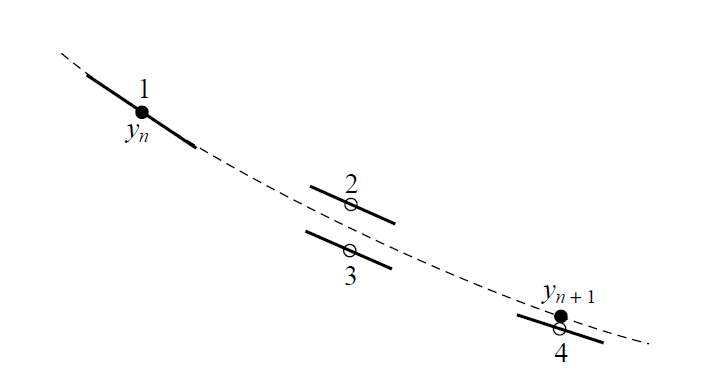Fourth-order Runge-Kutta method

The Runge-Kutta method treats every step in a sequence of steps identically. Prior behavior of a solution is not used in its propagation. This is mathematically proper since any point along the trajectory of an ordinary differential equation can serve as an initial point. All steps are treated identically and make it easy to incorporate Runge-Kutta into relatively simple "driver" schemes. The function 'ode45' is the same implementation of the described algorithm calculating the points in numerical order for our purpose as well.

## Simulating a free fall

The former case was a typical example of a second-order ordinary differential equation into action. The same procedure can be implemented for any other ODE and replace the initial conditions on the way. Now, we move to a procedure solving symbolic ODEs using the 'dsolve' function in MATLAB. Simulating a freefall on Earth is not as complex as the previous one. In this scenario, the change in the value of g (acceleration due to gravity can be taken as a constant throughout the process, simplifying the process. With the same ODE in hand, we simulate a condition where a ball is set to freefall from a height of 100m. Upon each impact, the ball loses 20% of the impact velocity due to inelastic collision. We try to render the animation till the max height reduces to only 5m. Let's look at the MATLAB code now.

clc
clear all
close all
syms y(t) %Declaring symbol
Dy = diff(y); %Differential of y
h = 100; %Initial height
g = 9.8; %Acceleration due to gravity
ode_y = diff(diff(y,t)) == -g; %Defining the ODE
condition = [Dy(0) == 0 y(0) == h]; %Adding initial values
ySol(t) = dsolve(ode_y, condition);
v = sqrt(2*g*h); %Finding the speed at which it will impact the ground
t_drop = v/g; %Finding the time taken to fall
while h>5 %Continues till bounce height is less than 5m
ts = linspace(0, t_drop, 100);
y_axis = ySol(t); %ODE solved eqution for specific bounce
x = 0;
for i = 1:length(ts)
k = plot(x,subs(y_axis,t,ts(i)),'r.','MarkerSize', 20); %plotting the bounce
axis([-2 2 0 100])
pause(0.01);
delete(k)
end
k = plot(x,0,'r.','MarkerSize', 20);
axis([-2 2 0 100])
v = 0.8*v; %Next Velocity is 80% of previous velocity
condition = [Dy(0) == v y(0) == 0]; %Initial conditions
ySol(t) = dsolve(ode_y, condition); %Solving ODE for next bounce

h = (v^2)/2*g; %Calculating max height of bounce
t_drop = 2*v/g; %Calculating Time of flight
end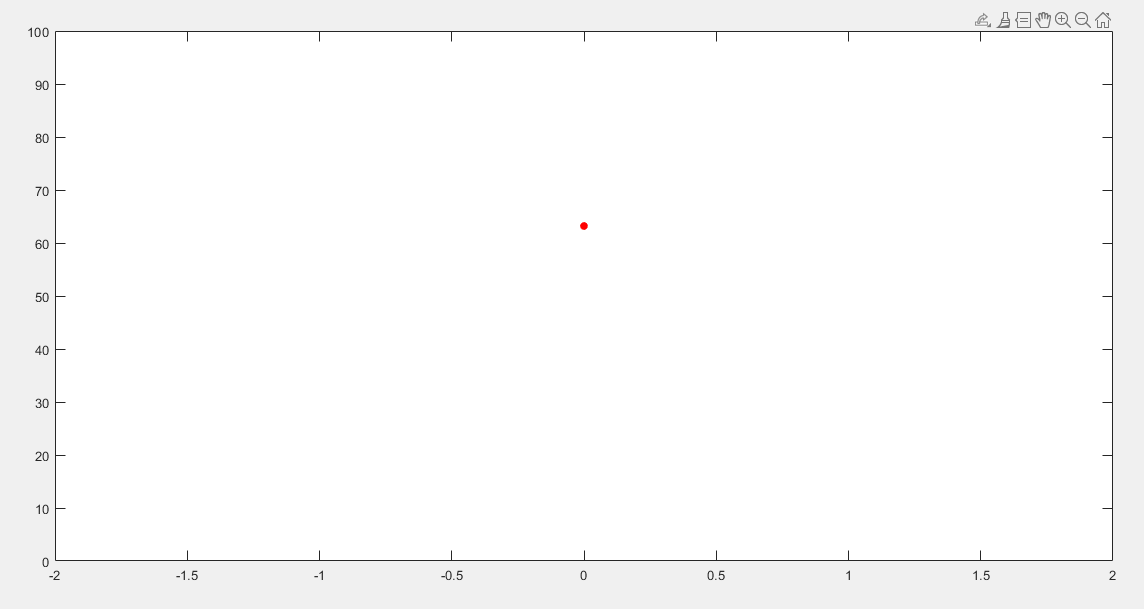Still Image of Freefall simulation

This case didn't use the numerical ODE solver and instead uses the symbolic solver where only two variables are considered. The height is progressing over time, and that's what the ODE solver tries to describe with the help of an equation.

## Conclusion & Discussion

As Physicists boast, Mathematics is the language of the Universe, and if they are correct, solving differential equations is a powerful tool for understanding the Universe. We went through two different approaches while solving the ordinary differential equation. Each has its own domain of operations application. It is now the person's discretion to use it to find which one is more suitable for the specific purpose.

Did you find some helpful content from our video or article and now looking for its code, model, or application? You can purchase the specific Title, if available, and instantly get the download link.

If you are looking for free help, you can post your comment below & wait for any community member to respond, which is not guaranteed. You can book Expert Help, a paid service, and get assistance in your requirement. If your timeline allows, we recommend you book the Research Assistance plan. If you want to get trained in MATLAB or Simulink, you may join one of our training modules.

If you are ready for the paid service, share your requirement with necessary attachments & inform us about any Service preference along with the timeline. Once evaluated, we will revert to you with more details and the next suggested step.

Education is our future. MATLAB is our feature. Happy MATLABing!

#### Soumya Shaw

Soumya Shaw is currently a graduated B.Tech student in Electronics & Communication from the Vellore Institute of Technology. He is an IEEE & SGAC member and interestingly working on Machine Learning, Image Processing and Digital Signal Processing. Besides, he is overzealous in the field of Cosmology, Higher Dimensional Mathematics & Quantum Mechanics.

#### Connect with MATLAB Helper ®

Use Website Chat or WhatsApp at +91-8104622179

Watch our Latest Video

Join a Training Module